# Class 9 NCERT Solutions- Chapter 8 Quadrilaterals – Exercise 8.1

• Difficulty Level : Basic
• Last Updated : 03 Jan, 2021

### Question 1. The angles of quadrilateral are in the ratio 3:5:9:13. Find all the angles of the quadrilateral.

Solution:

As we know that, the sum of the angles of a quadrilateral is 360° (Angle sum property of quadrilateral)

Hey! Looking for some great resources suitable for young ones? You've come to the right place. Check out our self-paced courses designed for students of grades I-XII

Start with topics like Python, HTML, ML, and learn to make some games and apps all with the help of our expertly designed content! So students worry no more, because GeeksforGeeks School is now here!

As they are in ratio 3 : 5 : 9 : 13, so we can assume angles be as 3x, 5x, 9x and 13x.

So,

3x + 5x + 9x + 13x = 360°

30x = 360°

x = 360/30 = 12°

So the angles will be as follows:

3x = 3×12 = 36°

5x = 5×12 = 60°

9x = 9×12 = 108°

13x = 13×12 = 156°

Hence, the angles of quadrilateral are 36°, 60°, 108° and 156°.

### Question 2. If the diagonals of a parallelogram are equal, then show that it is a rectangle.

Solution:

As mentioned parallelogram, so let PQRS be a parallelogram

where, given

PR = QS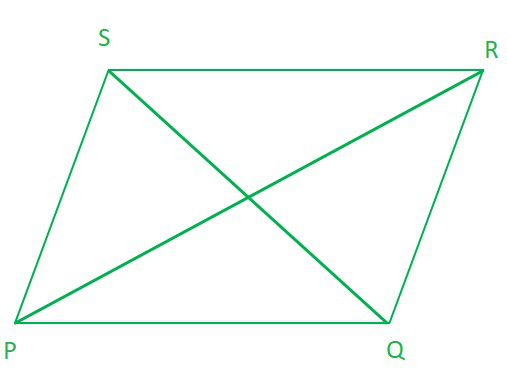In ∆PQR and ∆QRS,

PR = QS      …………………[Given]

PQ = RS      …………………[Opposite sides of a parallelogram]

QR = RQ     …………………[Common side]

∴ ∆PQR ≅ ∆QRS           [By SSS congruency]

so, ∠PQR = ∠QRS [By C.P.C.T.] ………………………………………….(1)

Now, PQ || RS and QR is a transversal. …………………….[PQRS is a parallelogram]

∴ ∠PQR + ∠QRS = 180°  [Co-interior angles of parallelogram]…………………………………… (2)

From (1) and (2), we have

∠PQR = ∠QRS = 90°

i.e., PQRS is a parallelogram having an angle equal to 90°.

Hence, PQRS is a rectangle. (having all angles equal to 90° and opposite sides are equal)

### Question 3. Show that if the diagonals of a quadrilateral bisect each other at right angles, then it is a rhombus.

Solution:

where, given

PO = RO

SO = QO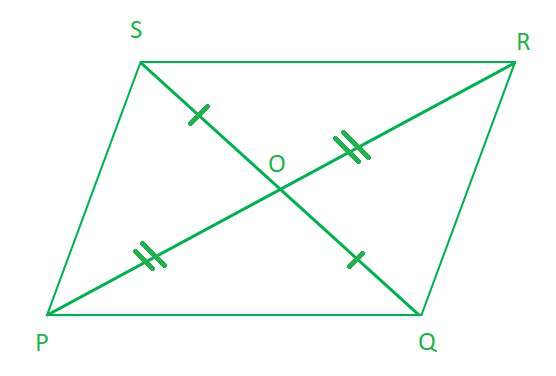In ∆POQ and ∆POS, we have

PO = PO [Common]

OQ = OS [O is the mid-point of QS]

∠POQ= ∠POS [Each 90°]

∴ ∆POQ ≅ ∆POS [By, SAS congruency]

∴ PQ = PS [By C.P.C.T.] ……..    (1)

Similarly, PQ = QR …………………..(2)

QR = RS ……………………………………..(3)

RS = SP ………………………………………(4)

∴ From (1), (2), (3) and (4), we have

PQ = QR = RS = SP

Thus, the quadrilateral PQRS is a rhombus.

Alternative Solution:

So as it is given that diagonals of a quadrilateral PQRS bisect each other

According to Theorem 8.7 NCERT it is a parallelogram

PQRS can be proved first a parallelogram then proving one pair of adjacent sides equal will result in rhombus.

In ∆POQ and ∆POS, we have

PO = PO [Common]

OQ = OS [O is the mid-point of QS]

∠POQ= ∠POS [Each 90°]

∴ ∆POQ ≅ ∆POS [By,SAS congruency]

∴ PQ = PS [By C.P.C.T.] ……..    (1)

Similarly, PQ = QR …………………..(2)

QR = RS ……………………………………..(3)

RS = SP ………………………………………(4)

From (1), (2), (3) and (4), we have

PQ = QR = RS = SP

Hence, as a parallelogram has all sides equal then it is called a rhombus.

### Question 4. Show that the diagonals of a square are equal and bisect each other at right angles.

Solution:

As it is mentioned that it is a square so,

all sides are equal. (PQ = QR = RS = SP)

all angles at four corners = 90°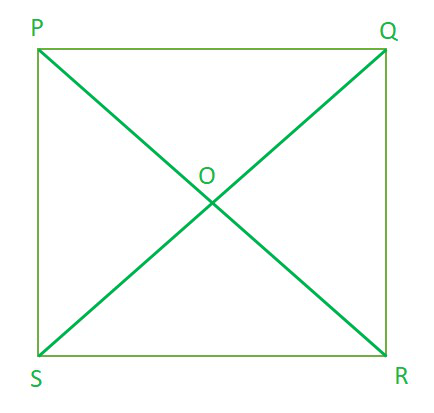So, to prove that the diagonals are equal, we need to prove PR = QS.

In ∆PQR and ∆QPS, we have

PQ = QP ……………….[Common]

PS = PQ ………………..[Sides of a square PQRS]

∠PQR = ∠QPS …….[Each angle is 90°]

∆PQR ≅ ∆QPS [By SAS congruency]

AC = BD [By C.P.C.T.] …(1)

Now as we know square is also a parallelogram

In ∆POQ and ∆ROS, we have

∠POQ = ∠ROS ……………….[Opposite angles of two intersecting lines]

PQ = RS ………………..[Sides of a square PQRS]

∠PQO = ∠RSO …….[Alternate interior angles are equal]

∆PQR ≅ ∆QPS [By ASA congruency]

OP = OQ = OR = OS (Hence the diagonals are equal and bisect each other)

Now , In ∆OQP and ∆OSP, we have

OQ = OS [Proved]

QP = SP [Sides of a square PQRS]

OP = OP [Common]

∆OQP ≅ ∆OSP [By SSS congruency]

∠POQ = ∠POS [By C.P.C.T.] …(3)

∠POQ + ∠POS = 180° (∵ ∠POQ and ∠POS form a linear pair)

∠POQ = ∠POS = 90° [By(3)]

PR ⊥ QS …(4)

From (1), (2) and (4), we get PR and QS are equal and bisect each other at right angles (90°).

### Question 5. Show that if the diagonals of a quadrilateral are equal and bisect each other at right angles, then it is a square.

Solution:

where,

PR = QS

OP = OR = OQ = OS

∠POQ = ∠QOR = ∠ROS = ∠SOP = 90°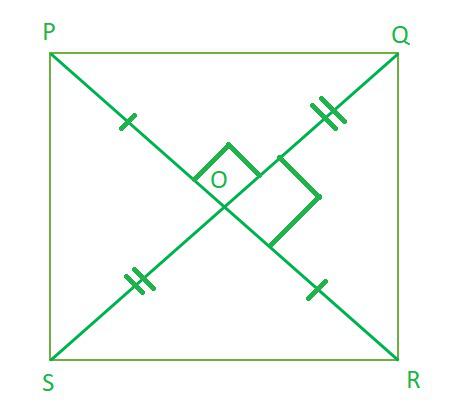Now, in ∆POS and ∆POQ, We have

∠POS = ∠POQ [Each 90°]

PO = PO [Common]

OS= OQ [ ∵ O is the midpoint of BD]

∆POS ≅ ∆POQ [By SAS congruency]

PS = PQ [By C.P.C.T.] …(1)

Similarly, we have

PQ = QR … (2)

QR = RS …(3)

RS = SP…(4)

From (1), (2), (3) and (4), we have

PQ = QR = RS = SP

Hence, Quadrilateral PQRS have all sides equal.

Now, In ∆POS and ∆ROQ, we have

PO = RO [Given]

OS = OQ [Given]

∠POS = ∠ROQ [Vertically opposite angles]

So, ∆POS ≅ ∆ROQ [By SAS congruency]

∠PSO = ∠RQO [By C.P.C.T.]

So as they form a pair of alternate interior angles.

PS || QR

Similarly, PQ || RS

PQRS is a parallelogram.

Parallelogram having all its sides equal is a rhombus.

PQRS is a rhombus.

Now, in ∆PQR and ∆QPS, we have

PR = QS [Given]

QR = SP [Proved]

PQ = QP [Common]

∆PQR ≅ ∆QPS [By SSS congruency]

∠PQR = ∠QPS [By C.P.C.T.] ……(5)

Since, PS || QR and PQ is a transversal.

∠PQR + ∠QPS = 180° .. .(6) [ Co – interior angles]

∠PQR = ∠QPS = 90° [By(5) & (6)]

So, rhombus PQRS is having corners angle equal to 90°.

Hence, PQRS is a square.

### Question 6. Diagonal AC of a parallelogram ABCD bisects ∠A (see Fig. 8.19). Show that

(i) it bisects ∠C also,

(ii) ABCD is a rhombus.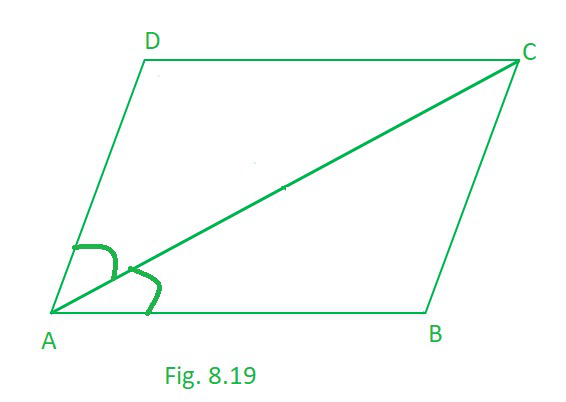Solution:

(i) As, ABCD is a parallelogram.

∠BAC = ∠DCA ……………………………(1) [Alternate interior angles are equal]

∠CAD = ∠BCA ………………………….(2) [Alternate interior angles are equal]

Also, ∠CAD = ∠CAB …………………….(3) [ (Given) as AC bisects ∠A]

From (1), (2) and (3), we have

∠DCA = ∠BCA

Hence, AC bisects ∠C.

(ii) In ∆ABC,

∠BAC = ∠DCA ………………………….. [Alternate interior angles are equal]

BC = AB …………………………….(4) [ Sides opposite to equal angles of a ∆ are equal]

But, ABCD is a parallelogram. [Given]

AB = DC ………………….(6) (opposite sides of parallelogram)

From (4), (5) and (6), we have

AB = BC = CD = DA

As, ABCD is a parallelogram having all sides equal then it is a rhombus.

### Question 7. ABCD is a rhombus. Show that diagonal AC bisects ∠ A as well as ∠ C and diagonal BD bisects ∠ B as well as ∠ D.

Solution:

As, ABCD is a rhombus, so

AB = BC = CD = DA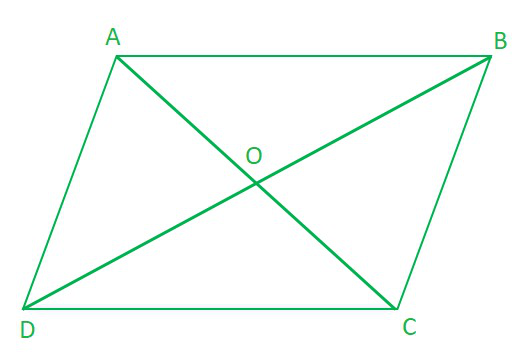∠ADB= ∠ABD……………………….(1) [Angles opposite to equal sides of a triangle are equal]

∠ADB = ∠CBD …………………..(2) [ ∵ Alternate interior angles are equal] (Rhombus is a parallelogram)

From (1) and (2), we have

∠CBD = ∠ABD …………………..(3)

∠ABD= ∠CDB ……………………..(4)      [ ∵ Alternate interior angles are equal]

From (1) and (4),

Hence, BD bisects ∠B as well as ∠D.

Similarly, we can prove that AC bisects ∠C as well as ∠A..

### Question 8. ABCD is a rectangle in which diagonal AC bisects ∠ A as well as ∠ C. Show that:

(i) ABCD is a square

(ii) diagonal BD bisects ∠ B as well as ∠ D.

Solution:

There is rectangle ABCD such that AC bisects ∠A as well as ∠C, so

∠BAC = ∠DAC and,

∠DCA = ∠BCA ………………………………(1)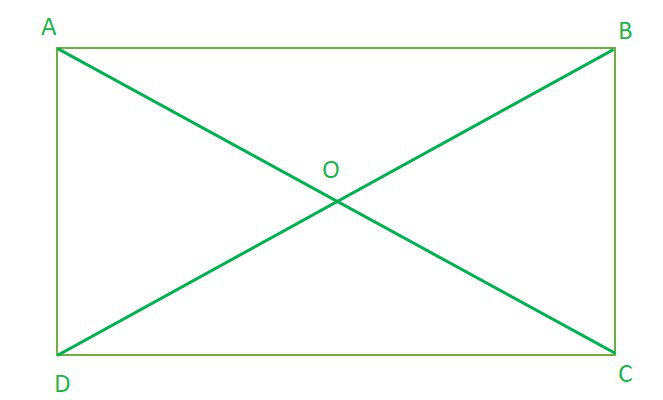(i) As we know that every rectangle is a parallelogram.

ABCD is a parallelogram.

∠BCA = ∠DAC …………………….(2) [ Alternate interior angles are equal]

From (1) and (2), we have

∠DCA= ∠DAC……………………….(3)

In ∆ABC, ∠DCA= ∠DAC then,

CD = DA [Sides opposite to equal angles of a ∆ are equal]

Similarly, AB = BC

So, ABCD is a rectangle having adjacent sides equal.

ABCD is a square.

(ii) Since, ABCD is a square

AB = BC = CD = DA

so, In ∆ABD, as AB = AD

∠ABD = ∠ADB [Angles opposite to equal sides of a ∆ are equal]……………………..(1)

Similarly, ∠CBD = ∠CDB…………………..(2)

∠CBD = ∠ADB               [Alternate interior angles are equal]………………(3)

From (1) and (3)

∠CBD = ∠ABD

From (2) and (3)

So, BD bisects ∠B as well as ∠D.

### Question 9. In parallelogram ABCD, two points P and Q are taken on diagonal BD such that DP = BQ (see Fig. 8.20). Show that :

(i) ∆APD ≅ ∆CQB

(ii) AP = CQ

(iii) ∆AQB ≅ ∆CPD

(iv) AQ = CP

(v) APCQ is a parallelogram

Solution:

ABCD is a parallelogram

DP = BQ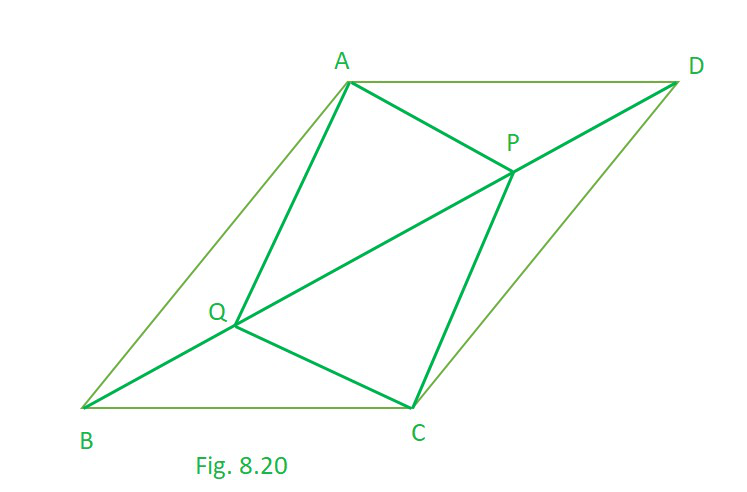(i) As ABCD is a parallelogram

∠ADB = ∠CBD [Alternate interior angles are equal]……………….(1)

∠ABD = ∠CDB   [Alternate interior angles are equal]…………………(2)

Now, in ∆APD and ∆CQB, we have

AD = CB [Opposite sides of a parallelogram ABCD are equal]

PD = QB [Given]

Hence, ∆APD ≅ ∆CQB [By SAS congruency]

(ii) As, ∆APD ≅ ∆CQB [Proved]

AP = CQ [By C.P.C.T.]…………………(3)

(iii) Now, in ∆AQB and ∆CPD, we have

QB = PD [Given]

∠ABQ = ∠CDP [Proved]

AB = CD [ Opposite sides of a parallelogram ABCD are equal]

Hence, ∆AQB ∆CPD [By SAS congruency]

(iv) As, ∆AQB ≅ ∆CPD [Proved]

AQ = CP [By C.P.C.T.] …………………………..(4)

Opposite sides are equal. [From (3) and (4)]

Hence, APCQ is a parallelogram. (NCERT Theorem 8.3)

### Question 10. ABCD is a parallelogram and AP and CQ are perpendiculars from vertices A and C on diagonal BD (see Fig. 8.21). Show that

(i) ∆APB ≅ ∆CQD

(ii) AP = CQ

Solution:

ABCD is a parallelogram

DP = BQ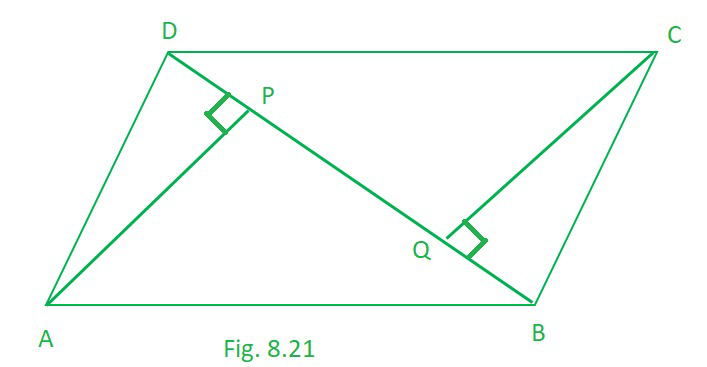(i) In ∆APB and ∆CQD, we have

∠APB = ∠CQD [Each 90°]

AB = CD [ Opposite sides of a parallelogram ABCD are equal]

∠ABP = ∠CDQ [Alternate angles are equal as AB || CD and BD is a transversal]

Hence, ∆APB  ≅  ∆CQD [By AAS congruency]

(ii) As, ∆APB ≅ ∆CQD [Proved]

AP = CQ [By C.P.C.T.]

### Question 11. In ∆ ABC and ∆ DEF, AB = DE, AB || DE, BC = EF and BC || EF. Vertices A, B and C are joined to vertices D, E and F respectively (see Fig. 8.22). Show that

(i) quadrilateral ABED is a parallelogram

(ii) quadrilateral BEFC is a parallelogram

(iv) quadrilateral ACFD is a parallelogram

(v) AC = DF

(vi) ∆ABC ≅ ∆DEF.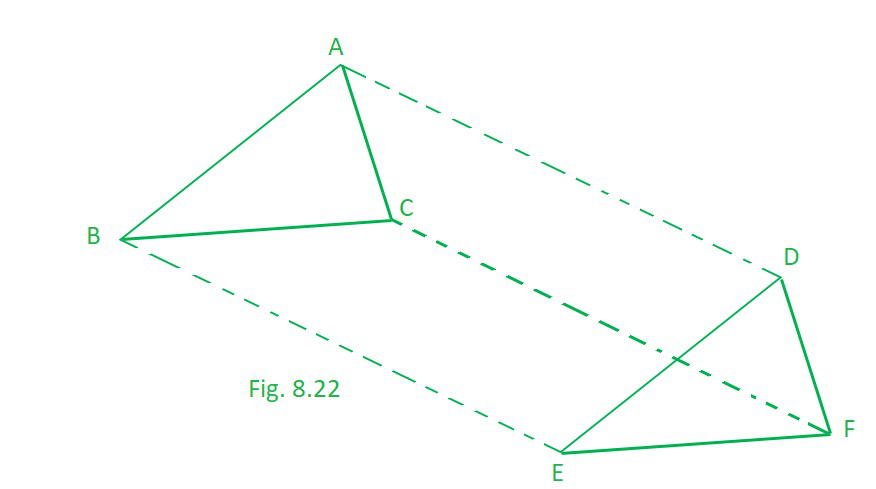Solution:

AB = DE, and AB || DE,

BC = EF and BC || EF

(i) We have AB = DE and AB || DE [Given]

so here., ABED is a quadrilateral in which a pair of opposite sides (AB and DE) are parallel and of equal length.

Hence, ABED is a parallelogram.

(ii) BC = EF and BC || EF [Given]

i.e. BEFC is a quadrilateral in which a pair of opposite sides (BC and EF) are parallel and of equal length.

Hence, BEFC is a parallelogram.

(iii) as, ABED is a parallelogram [Proved]

∴ AD || BE and AD = BE …(1) [Opposite sides of a parallelogram are equal and parallel]

Also, BEFC is a parallelogram. [Proved]

BE || CF and BE = CF …(2) [Opposite sides of a parallelogram are equal and parallel]

From (1) and (2), we have

i.e., In quadrilateral ACFD, one pair of opposite sides (AD and CF) are parallel and of equal length.

Hence, Quadrilateral ACFD is a parallelogram.

(v) Since, ACFD is a parallelogram. [Proved]

So, AC =DF [Opposite sides of a parallelogram are equal]

(vi) In ∆ABC and ∆DEF, we have

AB = DE [Given]

BC = EF [Given]

AC = DE [Proved in (v) part]

∆ABC ≅ ∆DEF [By SSS congruency]

### Question 12. ABCD is a trapezium in which AB || CD and AD = BC (see Fig. 8.23). Show that

(i) ∠A = ∠B

(ii) ∠C = ∠D

(iv) diagonal AC = diagonal BD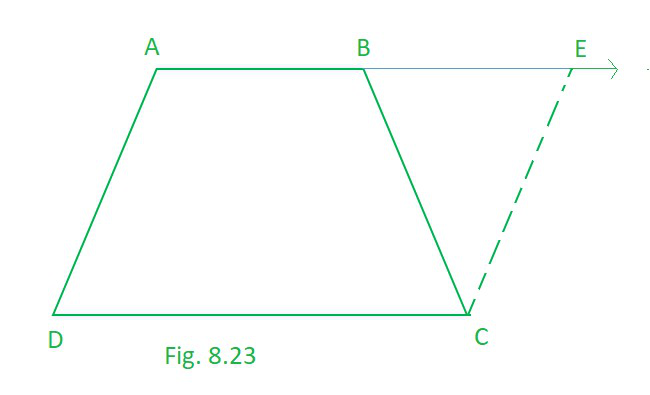[Hint: Extend AB and draw a line through C parallel to DA intersecting AB produced at E.]

Solution:

so basically here is a trapezium ABCD in which AB || CD and AD = BC.

Extended AB and draw a line through C parallel to DA intersecting AB produced at E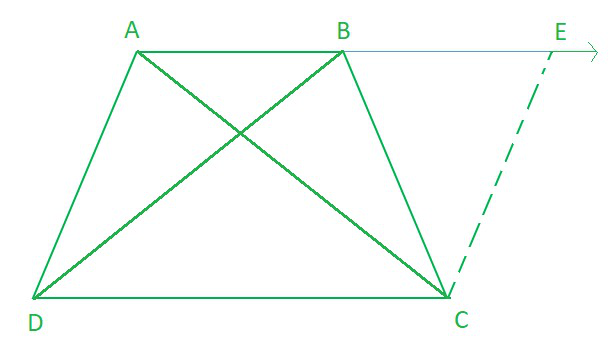(i) AB || DC , AE || DC Also AD || CE

then, AECD is a parallelogram.

AD = CE …………………………(1) [Opposite sides of the parallelogram are equal]

But AD = BC …(2) [Given]

From (1) and (2),

BC = CE

Now, in ∆BCF, we have BC = CF

∠CEB = ∠CBE …(3)  [Angles opposite to equal sides of a triangle are equal]

Also, ∠ABC + ∠CBE = 180° … (4) [Linear pair]

and ∠A + ∠CEB = 180° …(5) [Co-interior angles of a parallelogram ADCE]

From (4) and (5), we get

∠ABC + ∠CBE = ∠A + ∠CEB

∠ABC = ∠A [From (3)]

∠B = ∠A …(6)

(ii) AB || CD and AD is a transversal.

∠A + ∠D = 180° …(7) [Co-interior angles in parallelogram]

Similarly, ∠B + ∠C = 180° … (8)

From (7) and (8), we get

∠A + ∠D = ∠B + ∠C

∠C = ∠D [From (6)]

(iii) In ∆ABC and ∆BAD, we have

AB = BA [Common]